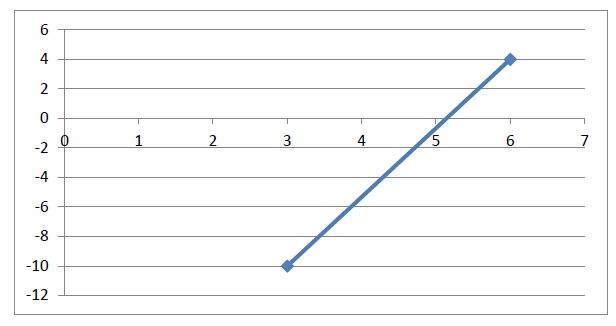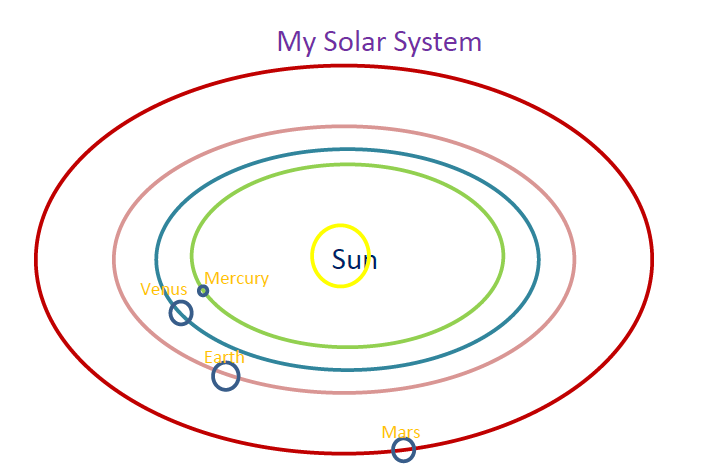# Week 3 Homework – CMSC405

35.00 \$ 17.50 \$

Categories: ,
Click Category Button to View Your Next Assignment | Homework

You'll get a: . ` zip` file solution, download link after Payment

## Description

1. Given the 2 end points shown on the graph below, determine the slope, y-intercept and give the slope-intercept formula. Determine one additional point that would be on that line using the slope-intercept formula where the x value would be greater than 6.Be sure to show your work.
2. Calculate the plane coefficients (A,B,C and D) of 3 points in a plane defined by P1, P2 and P3, and determine if the point P4 is behind or in front of the polygon surface contained within that plane:
P1 = (10,-20,40); P2 = (30,3,18); P3 = (-5,-10,25); P4 = (-10,12,-19);
Be sure to show your work. Explain why determining if Point P4 is behind or in front of the polygon surface relevant to computer graphics applications.
3. Using Visual C++ and your OpenGL configured environment, write an application that displays a graphical scene that displays a top-down, 2-D (non-animated) view of the Sun and at least 4 planets of our solar system. You can assume elliptical orbits for all of the planets in your scene. Sizes of the ellipses should be scaled to the actual distances from the Sun. You should include circles representing each of the planets and the Sun in your scene. Sizes of the objects should be scaled proportional to their actual size (with the exception of the Sun as it will be most likely too large to have other planet be visible). Be sure to label each of your planets and the Sun. In the word document you submit, describe exactly how you scaled your planets and elliptical orbits. Be sure to reference your source for planet size, and distance from the Sun. (e.g. http://www.solarviews.com/eng/solarsys.htm)
-12
-10
-8
-6
-4
-2
0
2
4
6
0
1
2
3
4
5
6
7
2
A “rough” graphics scene might look like this:Using Visual C++ and your OpenGL configured environment, write an application that calculates and plots the 2D parabolic trajectories for an object whose starting position is p1 = (500,400) with velocities, v = (30m/s,40 m/s), for at least 4 different planets including Earth. Plot the trajectories using different line colors and styles for values of time increments of 1 second. Plot the trajectories only up to the first negative Y value. For example, the last 3 plotted points (x,y) might be
860
174.4
890
91.9
920
-0.4
No additional points beyond the first negative value should be plotted.
Label the trajectories corresponding to the planets selected. Be sure to reference your source used for the gravity for the planets. Be sure your plot is well-organized, includes grid lines, x and y axis, x-axis and y-axis labels, and legends. Each trajectory should use a different symbol or shape or color. One possible graphic result is shown below.